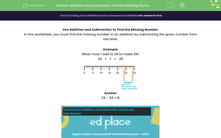# Solve Addition Problems Using a Number Line

In this worksheet, students will explore the relationship between addition and subtraction to help them find missing numbers.Key stage:  KS 1

Curriculum topic:   Number: Addition and Subtraction

Curriculum subtopic:   Understand Relationship Between Addition and Subtraction

Difficulty level:#### Worksheet Overview

In this activity, we will be finding the missing number in an addition.

Example

What must I add to 24 to make 29?

24   +   ?   =   29Place your finger on 29 and bounce it back, to the left,  one number at a time, until you reach 25. How many bounces did you do? It was 5 bounces, so we know that 29 − 24 = 5

We could also count forwards, instead of back, if we wanted. Pop your finger on 24 and bounce it to the right, one number at a time, until you reach 29. How many bounces did you do? It was 5 again.

So, 24 + 5 = 29

There is often more than one way to solve a problem in maths. Why not try both methods and see which one works best for you?### What is EdPlace?

We're your National Curriculum aligned online education content provider helping each child succeed in English, maths and science from year 1 to GCSE. With an EdPlace account you’ll be able to track and measure progress, helping each child achieve their best. We build confidence and attainment by personalising each child’s learning at a level that suits them.

Get started